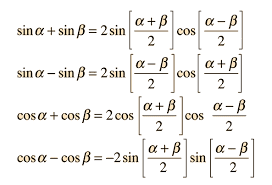# Adding trig functions with different amplitudes

• I
• Mayhem
In summary, the conversation discusses the use of trig identities in adding trig functions and their application in a Fourier transform. It is mentioned that there is no general simplification for situations where there are two arbitrary, real amplitudes for each term, and a workaround may be needed. The conversation also touches on the Fast Fourier Transformation algorithm and the derivation of trig identities for turning products into sums. It is suggested to use these identities in a Fourier transform, but it is unclear why one would want to do so instead of using the inverse Fourier transform.f

#### Mayhem

TL;DR Summary
A general rule for adding two trigonometric functions that have unidentical amplitudes
The trig identities for adding trig functions can be seen:But here the amplitudes are identical (i.e. A = 1). However, what do I do if I have two arbitrary, real amplitudes for each term? How would the identity change?

Analysis: If the amplitudes do show up on the RHS, we would expect them to either be a product or sum of these, possibly signed, or simply explicitly states. For A = 1, it may be difficult to see where they appear if explicitly stated, as they disappear as a factor. However, what we do see is that 2 appears in front of all of the RHS identities, which is a hint that for A_1 = A_2 = 1, we simply add them together and place them as a factor in front of the expression. However, this isn't necessarily the case, and simply and intuition, and considering 2 also appears in the denominators of the inner terms, it isn't a given that the number 2 shows up for this reason.

I don't know the derivations of the above identities, so I'm wondering if there is a way to generalize something like Asin(a) + Bsin(b) such that these terms are accounted for on the RHS of an equivalent expression.

There is no general simplification for that situation. You just have to leave them as the multiplied sum of two different trig functions.

There is no general simplification for that situation. You just have to leave them as the multiplied sum of two different trig functions.
Well that's annoying. The problem is I am trying to program a Fourier transform, which requires me to make a linear combination of trig functions. However, I can probably figure out a workaround.

Well that's annoying. The problem is I am trying to program a Fourier transform, which requires me to make a linear combination of trig functions. However, I can probably figure out a workaround.
If your program only has to calculate a linear combination of a couple of trig functions, then a computer can easily do the calculation directly.
If you are trying to calculate the Fourier transform of a general function and have enough data points, then you should look into the Fast Fourier Transformation (FFT). There are several implementations of the FFT algorithm. (see https://en.wikipedia.org/wiki/Fast_Fourier_transform)

Last edited:
•topsquark
These identities are derived from $$\begin{split} \cos(a \pm b) &= \cos a \cos b \mp \sin a \sin b, \\ \sin(a \pm b) &= \sin a \cos b \pm \cos a \sin b. \end{split}$$ Their purpose in the context of transforms is to turn products into sums. For example $$\begin{split} \left(\sum_{n=0}^N a_n \cos(nx)\right)\left(\sum_{n=0}^N b_n \cos(nx)\right) &= \sum_{n=0}^N \sum_{m=0}^N a_n b_m \cos (nx) \cos(m x) \\ &= \frac12 \sum_{n=0}^N \sum_{m=0}^N a_nb_m \left( \cos((n+m)x) + \cos((n-m)x)\right)\end{split}$$ and from there you can work out which values of $n$ and $m$ will contribute to the coefficients $c_r$ in $\sum_{r=0}^N c_r \cos(rx)$.

You can use these identities to do what you were originally attempting, but it is unclear to me why you would want to write $(A + B)\sin((a+b)/2)\cos((a-b)/2) + (A - B)\cos((a+b)/2)\sin((a-b)/2)$ instead of $A \sin a + B \sin b$.

Last edited:
•PeroK, Mayhem and topsquark